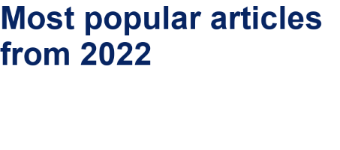# Cooper pair splitting controlled by a temperature gradient

1QTF Centre of Excellence, Department of Applied Physics, Aalto University, FI-00076 Aalto, Finland
2I.E. Tamm Department of Theoretical Physics, P.N. Lebedev Physical Institute, 119991 Moscow, Russia
3National Research University Higher School of Economics, 101000 Moscow, Russia

1. Corresponding author email

Guest Editor: A. S. Sidorenko
Beilstein J. Nanotechnol. 2023, 14, 61–67. https://doi.org/10.3762/bjnano.14.7
Received 01 Oct 2022, Accepted 23 Dec 2022, Published 09 Jan 2023

• Full Research Paper

## Abstract

Electrons in two different normal metallic electrodes attached to a sufficiently thin superconducting island may become entangled due to the effect of Cooper pair splitting. This phenomenon is of fundamental importance and may also have serious implications for developing quantum communication technologies. One way to identify Cooper pair splitting is to analyze long-range cross correlations of fluctuating currents in three-terminal hybrid normal–superconducting–normal nanostructures. Here, we theoretically investigate non-trivial behavior of cross-correlated non-local shot noise in the presence of a temperature gradient. We suggest that applying a temperature gradient may serve as an extra tool to control the phenomenon of Cooper pair splitting.

Keywords: Cooper pair splitting; entanglement; quantum shot noise; superconducting hybrid nanostructures

## Introduction

Normal metals connected to a superconductor exhibit a variety of non-trivial phenomena associated with the existence of proximity-induced superconducting correlations spreading over long distances at sufficiently low temperatures . One of these phenomena is the so-called crossed Andreev reflection (CAR): A Cooper pair may split into two electrons  (see Figure 1a), thereby generating pairs of entangled electrons in different metallic electrodes . This phenomenon and its effect on electron transport in normal metal–superconductor–normal metal (NSN) hybrid structures were intensively investigated both theoretically [4-10] and experimentally [11-18] over the past decades.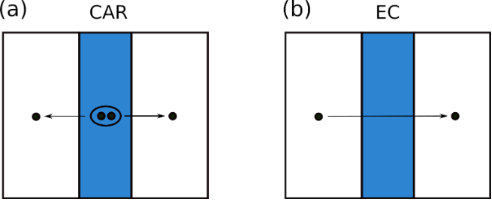Figure 1: Schematics of the processes of crossed Andreev reflection (a) and elastic cotunneling (b). These schemes are redrawn from . Figure 1: Schematics of the processes of crossed Andreev reflection (a) and elastic cotunneling (b). These sc... Jump to Figure 1

The process competing with CAR is the so-called elastic cotunneling (EC), where an electron is transferred from one normal metal to another across an effective barrier created by the energy gap inside the superconductor, see Figure 1b. Unlike CAR, EC does not produce entangled electrons. In the zero-temperature limit, CAR and EC contributions to the low-bias non-local conductance of an NSN device cancel each other in the limit of low-transparency barriers . In contrast, at high transmissions, the CAR contribution vanishes [6,7]. These observations make an unambiguous identification of CAR in transport experiments a non-trivial task.

The way out is to investigate fluctuations of electric currents passing through both NS boundaries of an NSN structure. While in non-superconducting multiterminal structures cross correlations of current noise in different terminals always remain negative , such cross correlations may become positive in the presence of superconductivity due to the process of CAR. This conclusion was initially reached theoretically in the limit of low-transparency barriers at NS interfaces [20,21] and later extended to the case of arbitrary barrier transmissions [22-25]. Positively cross-correlated non-local shot noise was indeed observed in a number of experiments [26,27]. Real-time observation of Cooper pair splitting was also reported in a recent work .

Usually, an interplay between positive and negative cross correlations of current noise in NSN devices can be controlled and tuned by applying external bias voltages. In this work we suggest another way of controlling Cooper pair splitting: We predict and investigate non-trivial behavior of cross-correlated non-local shot noise in the presence of a temperature gradient. Note that, previously, this so-called “delta-T” noise was studied in normal atomic-scale junctions . Here, we demonstrate that such kind of noise can also manifest itself in subtle non-local properties of hybrid NSN structures associated with the phenomena of crossed Andreev reflection and Cooper pair splitting.

## Results and Discussion

Let us consider the NSN structure depicted in Figure 2. Normal metallic leads are attached to a bulk superconductor with the aid of two junctions described by a set of conducting channel transmissions τ1,n and τ2,n with n being the integer number enumerating all conducting channels. The two junctions are located at a distance considerably shorter than the superconducting coherence length ξ. Normal electrodes are kept at different temperatures T1 and T2, thus creating a temperature gradient across our device. In addition, voltages V1 and V2 can be applied to two normal leads, as shown in Figure 2.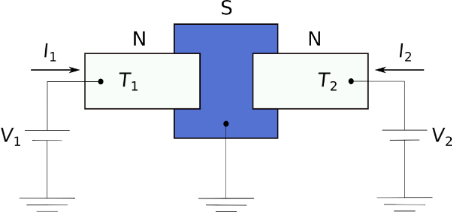Figure 2: Schematics of the NSN structure under consideration. Normal electrodes are biased by external voltages V1 and V2 and are kept at different temperatures T1 and T2. The superconducting electrode is assumed to be thinner than the superconducting coherence length ξ. Figure 2: Schematics of the NSN structure under consideration. Normal electrodes are biased by external volta... Jump to Figure 2

The Hamiltonian of this structure can be chosen in the form

where

are the Hamiltonians of two normal leads,denote creation and annihilation operators for an electron with a spin projection α at a point x, m is the electron mass, and μ is the chemical potential,

is the Hamiltonian of a superconducting electrode with the order parameter Δ and the terms

account for electron transfer through the junctions between the superconductor and the normal leads. In Equation 4, the surface integrals are taken over the contact areas, and tr(x) denote coordinate- and spin-independent tunneling amplitudes.

Let us denote the probability for N1 and N2 electrons to be transferred, respectively, through the junctions 1 and 2 during the observation time t as Pt(N1,N2). Introducing the so-called cumulant generating function12) by means of the formula

with χ1,2 being the counting fields, one can express the average currents through the junctions, and the current–current correlation functions

in the following form

The cumulant generating functionin Equation 5 be evaluated in a general form with the aid of the path integral technique [22,25], which yields

whereis the Keldysh Green function of our system

the 4 × 4 matricesrepresent the inverse Keldysh Green functions of isolated normal and superconducting leads andis the diagonal 4 × 4 matrix in the Nambu–Keldysh space describing tunneling between the leads,

Further analysis of the general expression for the function(Equation 8) is essentially identical to that already carried out in . Therefore, it is not necessary to go into details here. Employing Equation 7 and making use of the results , we recover general expressions for both the currents Ir across the junctions and the cross-correlated current noise S12 in the presence of a temperature gradient between two normal terminals.

In what follows we will be particularly interested in the limit of low voltages and temperatures eV1,2,T1,2 ≪ Δ. In this case, Ir (containing both local and non-local components) is practically insensitive to temperature and matches with the results [6,7,10] derived earlier in the corresponding limit.

For the non-local current noise in the same limit eV1,2,T1,2 ≪ Δ we obtain

where we defined the Andreev reflection probabilities per conducting channel in both junctions

introduced the function

and employed Fermi distribution functions for electrons and holes in the normal leads

Equation 11 defines the low-energy cross-correlated current noise in the presence of a temperature gradient and represents the main general result of the present work.

The expression (Equation 11) contains the integrals of the type, which cannot be handled analytically except in some special limits, i.e.,

for T1 = T2 = T and

for T1T2. In the opposite limit T2T1 in Equation 16 one should simply interchange T1T2.

In order to proceed, we note that there exists a very accurate interpolation formula between the above limits. It reads

where we defined an effective temperature

and introduced the notations T = (T1 + T2)/2 and δT = T1T2.

With the aid of this interpolation, the non-local noise (Equation 11) can be reduced to a much simpler form

Here we have introduced the non-local conductance in the limit of zero temperature and zero bias voltage,being the normal state resistance of a superconducting island , as well as the parameters

and the local Fano factors for two barriers in the Andreev reflection regime

At zero bias voltages V1 = V2 = 0, we obtain the noise in the form

Hence, for the excess non-local noise δS12 = S12(TT) − S12(T,0) induced by the temperature gradient we get

This contribution is positive and reaches its maximum δS12 ≃ 0.44G12T at |δT| = T.

The effect of the temperature gradient on cross-correlated non-local noise remains appreciable also at non-zero bias voltages V1,2, in which case it essentially depends on transmission distributions in both junctions.

We start from the tunneling limit A1,n, A2,m ≪ 1, where one has

Keeping only the terms ∝γ± in the expression (Equation 19), we obtain

The first and the second terms on the right-hand side of this formula are attributed, respectively, to CAR and EC processes. We observe that, similarly to the limit δT = 0, the noise cross correlations remain positive, S12 > 0, provided V1 and V2 have the same sign, and they turn negative, S12 < 0, should V1 and V2 have different signs. The result is also illustrated in Figure 3.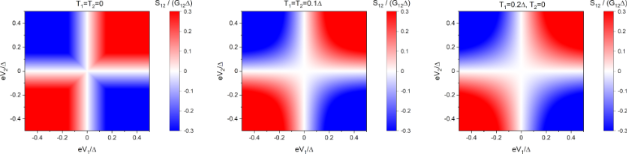Figure 3: Non-local noise S12 (Equation 26) in the tunneling limit (Equation 25). Left panel: T = 0; middle panel: T = 0.1Δ, δT = 0; right panel: |δT| = T = 0.1Δ. Figure 3: Non-local noise S12 (Equation 26) in the tunneling limit (Equation 25). Left panel: T = 0; middle panel: T = 0.1Δ, δT = 0... Jump to Figure 3

In the opposite limit of perfectly conducting channels in both junctions with τ1,n = τ2,m = 1 one gets γ+ = 2, γ = 0, β1 = β2 = 0. Hence, in this case, Equation 19 yields

This result is always positive at non-zero bias and sufficiently low temperatures, indicating the importance of CAR processes in this limit, see also Figure 4.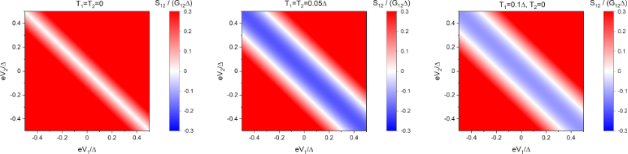Figure 4: Non-local noise S12 (Equation 27) in the case of fully transparent junctions. Left panel: T = 0; middle panel: T = 0.05Δ, δT = 0; right panel: |δT| = T = 0.05Δ. Figure 4: Non-local noise S12 (Equation 27) in the case of fully transparent junctions. Left panel: T = 0; middle panel: ... Jump to Figure 4

Yet another important physical limit is realized provided the contact has the form of a short diffusive wire with the corresponding Thouless energy exceeding the superconducting gap Δ. In this diffusive limit the transmission probability distributions in both junctions are defined by the well-known formula

withbeing the resistances of diffusive contacts in the normal state. Making use of this formula, one readily finds γ± = ±1 and β1 = β2 = 1/3. Then Equation 19 reduces to

This result is also displayed in Figure 5.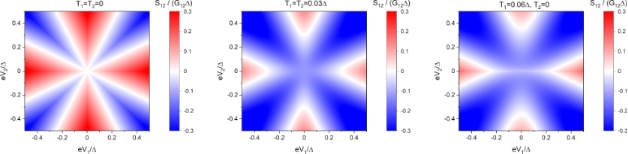Figure 5: Non-local noise S12 (Equation 29) in the case of diffusive barriers. Left panel: T = 0; middle panel: T = 0.03Δ, δT = 0; right panel: |δT| = T = 0.03Δ. Figure 5: Non-local noise S12 (Equation 29) in the case of diffusive barriers. Left panel: T = 0; middle panel: T = 0.03... Jump to Figure 5

## Conclusion

Comparing the non-local shot noise pattern in all the above limits, we can make several important conclusions. First, in full accordance with our previous results [22,25], this pattern turns out to be markedly different depending on particular transmission distributions for intermetallic barriers, thus emphasizing different roles played by CAR and EC processes. Second, relative contributions of the latter processes can be reliably controlled by applied external bias voltages V1 and V2 as well as by varying temperature T. Third, we observe that the non-local shot noise patterns undergoes additional modifications provided a temperature gradient is applied to our structure. Hence, the temperature gradient, along with other parameters, can also serve as a possible extra tool to control and tune the process of Cooper pair splitting in multiterminal hybrid normal–superconducting metallic structures.

#### Article is part of the thematic issue

##### Intrinsic Josephson effect and prospects of superconducting spintronics

Anatolie S. Sidorenko, Vladimir Krasnov and Horst Hahn

#### Interesting articles

##### A superconducting adiabatic neuron in a quantum regime

Marina V. Bastrakova, Dmitrii S. Pashin, Dmitriy A. Rybin, Andrey E. Schegolev, Nikolay V. Klenov, Igor I. Soloviev, Anastasiya A. Gorchavkina and Arkady M. Satanin

##### Plasma modes in capacitively coupled superconducting nanowires

Alex Latyshev, Andrew G. Semenov and Andrei D. Zaikin

##### Superconductor–insulator transition in capacitively coupled superconducting nanowires

Alex Latyshev, Andrew G. Semenov and Andrei D. Zaikin

#### News

##### Beilstein Nanotechnology Symposium 2023 - May 9 to 11, 2023, Limburg, Germany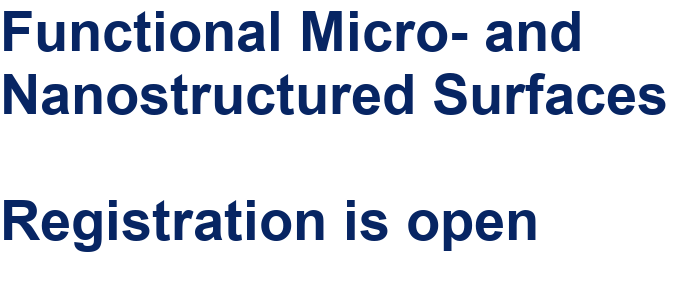##### Online Beilstein Talk with Antonio Abate on February 2 – Register for free!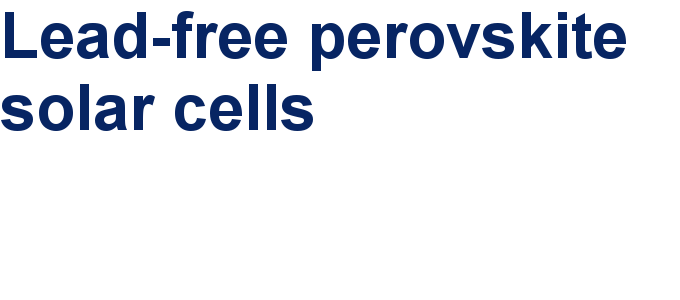##### Take a look at the research you were most interested in last year